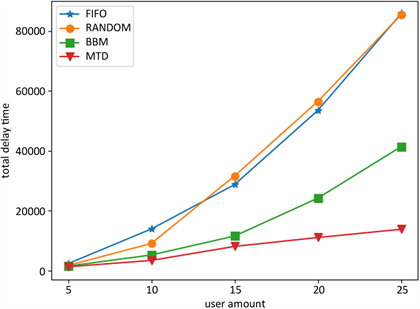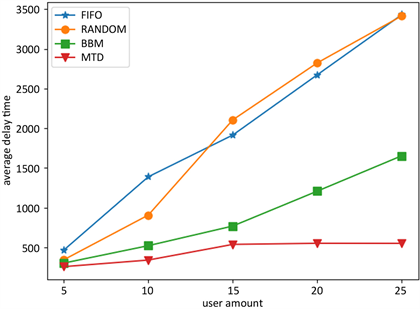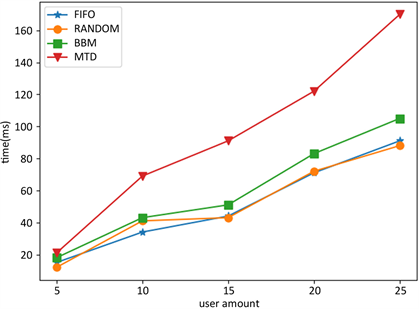# 一种移动边缘计算中最小总滞后时间的调度算法A Scheduling Algorithm for Minimum Total Delay Time in Mobile Edge Computing

DOI: 10.12677/SEA.2019.86036, PDF, HTML, XML, 下载: 294  浏览: 632

Abstract: As an emerging architecture, mobile edge computing extends cloud computing services to the edge of the network close to users through mobile edge computing servers, meeting the needs of appli-cations that require real-time control and real-time data analysis. However, due to the limited computing power of the mobile edge computing server, the delay time of the task is long. In order to improve the status quo, this paper proposes a scheduling algorithm with minimum total delay time. The server determines the optimal order of task calculation to minimize the total lag time. In addition, this paper also proposes an incentive mechanism that allows users to submit tasks with reasonable computational effort and expected completion time, while reducing the number and amount of submitted tasks when the server computing resources are insufficient. The results show that the proposed algorithm’s performance is close to the traditional scheduling algorithms, and increases 17% to 200% in total delay time and average delay time.

1. 引言

2. 问题描述Figure 1. Framework of mobile edge computing

$\begin{array}{cc}\mathrm{min}& \underset{i=0}{\overset{n}{\sum }}{l}_{i}\\ \text{s}\text{.t}\text{.}& {f}_{i}\le \underset{i=0}{\overset{n}{\sum }}{p}_{i},\forall i\\ & {s}_{i}+{p}_{i}={f}_{i},\forall i\end{array}$ (1)

3. 调度算法

1) 某种顺序的任务排列： $\left\{{t}_{0},{t}_{1},\cdots ,{t}_{k-1},{t}_{k+1},\cdots ,{t}_{k+\delta }\right\}$

2) 任务 ${t}_{k}$

3) 某种顺序的任务排列： $\left\{{t}_{k+\delta +1},{t}_{k+\delta +2},\cdots ,{t}_{n}\right\}$

${f}_{k}\left(\delta \right)=\underset{j\le k|\delta }{\sum }{p}_{j}$ (2)

$V\left(\varnothing ,\gamma \right)=0$ (3)

$V\left(\left\{j\right\},\gamma \right)=\mathrm{max}\left(0,\gamma +{p}_{j}-{d}_{j}\right)$ (4)

$\begin{array}{c}V\left(T\left(j,n,k\right),t\right)=\mathrm{min}\left(V\left(j,{k}^{\prime }+\delta ,{k}^{\prime }\right),\gamma \right)+\mathrm{max}\left(0,{f}_{{k}^{\prime }}\left(\delta \right)-{d}_{{k}^{\prime }}\right)\\ \text{\hspace{0.17em}}\text{\hspace{0.17em}}+V\left(T\left({k}^{\prime }+\delta +1,n,{k}^{\prime }\right),{f}_{{k}^{\prime }}\left(\delta \right)\right)\end{array}$ (5)

${p}_{{k}^{\prime }}=\mathrm{max}\left({p}_{{j}^{\prime }}|{j}^{\prime }\in T\left(j,n,k\right)\right)$ (6)

$V\left(\left\{{t}_{0},{t}_{1},\cdots ,{t}_{n}\right\},0\right)$ (7)

4. 激励机制

${C}_{i}=\alpha {p}_{i}\cdot {\beta }^{{d}_{i}-{p}_{i}}\cdot {\text{e}}^{\gamma P}$ (8)

5. 实验与性能分析

5.1. 实验设置

5.2. 结果分析Figure 2. Comparison of total delay timeFigure 3. Comparison of average delay timeFigure 4. Comparison of scheduling order calculation time

6. 结语